##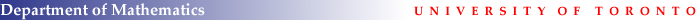### CORE COURSES

MAT 1000HF (MAT 457Y1Y)
REAL ANALYSIS I
L. Guth

Measure Theory: Lebesque measure and integration, convergence theorems, Fubini's theorem, Lebesgue differentiation theorem, abstract measures, Caratheodory theorem, Radon-Nikodym theorem.

Functional Analysis: Hilbert spaces, orthonormal bases, Riesz representation theorem, compact operators, Lspaces, Holder and Minkowski inequalities.

Textbooks:
Elias Stein and Rami Shakarchi, Measure Theory, Integration, and Hilbert Spaces,

References:
H.L. Royden: Real Analysis, Macmillan, 1988.

G. Folland, Real Analysis: Modern Techniques and their Applications, Wiley
A.N. Kolmogorov and S.V. Fomin: Introductory Real Analysis, 1975.

MAT 1001HS (MAT 457Y1Y)
REAL ANALYSIS II
L. Guth

Fourier analysis: Fourier series and transform, convergence results, Fourier inversion theorem, L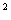theory, estimates, convolutions.

More functional analysis:  Banach spaces, duals, weak topology, weak compactness, Hahn-Banach theorem, open mapping theorem, uniform boundedness theorem.

Textbooks:
Elias Stein and Rami Shakarchi, Measure Theory, Integration, and Hilbert Spaces,

References:
G. Folland, Real Analysis: Modern Techniques and their Applications, Wiley.
S.D. Promislow, A First Course in Functional Analysis, Wiley, 2008.

A.N. Kolmogorov and S.V. Fomin: Introductory Real Analysis, 1975.

MAT 1002HS (MAT 454H1S)
COMPLEX ANALYSIS
C. Pugh

1. Review of elementary properties of holomorphic functions. Cauchy's integral formula, Taylor and Laurent series, residue calculus.
2. Harmonic functions. Poisson's integral formula and Dirichlet's problem.
3. Conformal mapping, Riemann mapping theorem.
4. Analytic continuation, Monodromy Theorem, Riemann surfaces.
5. Modular functions and the Picard Theorems.
6. Other topics are possible, like product theorems, elliptic functions, and non-isolated removability theorems.

Main References:
Stein and Shakarch: Complex Analysis
L. Ahlfors: Complex Analysis, 3rd Edition, McGraw-Hill, New York, 1966.

W. Rudin: Real and Complex Analysis, 2nd Edition, McGraw-Hill, New York,
1974.
Remmert: Theory of Complex Functions
Remmert: Classical topics in Complex function theory
Garnett and Marshall: Harmonic Measure
Needham: Visual Complex Analysis
Bierstone:  Notes taken by Oleg Ivrii

MAT 1060HF
PARTIAL DIFFERENTIAL EQUATIONS I
A. Burchard

This is a basic introduction to partial differential equations as they arise in physics, geometry and optimization. It is meant to be accessible to beginners with little or no prior knowledge of the field. It is also meant to introduce beautiful ideas and techniques which are part of most analysts' basic bag of tools. A key theme will be the development of techniques for studying non-smooth solutions to these equations.

Textbook:
L.C. Evans, "Partial Differential Equations"

MAT 1061HS
PARTIAL DIFFERENTIAL EQUATIONS II
J. Colliander

This course will consider a range of mostly nonlinear partial differential equations, including elliptic and parabolic PDE, as well as hyperbolic and other nonlinear wave equations. In order to study these equations, we will develop a variety of methods, including variational techniques, and fixed point theorems.  One important theme will be the relationship between variational questions, such as critical Sobolev exponents, and issues related to nonlinear evolution equations, such as finite-time blowup of solutions and/or long-time asymptotics.
The prerequisites for the course include familiarity with Sobolev and other function spaces, and in particular with fundamental embedding and compactness theorems.
Other topics in PDE will also be discussed.

Reference:
Lawrence Evans: Partial Differential Equations

MAT 1100HF
ALGEBRA I
S. Arkhipov

Basic notions of linear algebra: brief recollection. The language of Hom spaces and the corresponding canonical isomorphisms. Tensor product of vector spaces.

Group Theory: Isomorphism theorems, group actions, Jordan-Hölder theorem, Sylow theorems, direct and semidirect products, finitely generated abelian groups, simple groups, symmetric groups, linear groups, nilpotent and solvable groups, generators and relations.

Ring Theory: Rings, ideals, Euclidean domains, principal ideal domains, and unique factorization domains.

Modules: Modules and algebras over a ring, tensor products, modules over a principal
ideal domain,

Textbooks:
Dummit and Foote: Abstract Algebra, 2nd Edition
Lang: Algebra, 3rd Edition.

Other References:
Jacobson: Basic Algebra, Volumes I and II.
Cohn: Basic Algebra
M. Artin: Algebra.

MAT 1101HS
ALGEBRA II
S. Arkhipov

Fields: Algebraic and transcendental extensions, normal and separable extensions, fundamental theorem of Galois theory, solution of equations by radicals.

Commutative Rings: Noetherian rings, Hilbert basis theorem, invariant theory, Hilbert Nullstellensatz, primary decomposition, affine algebraic varieties. structure of semisimple algebras, application to representation theory of finite groups.

Textbooks:
Dummit and Foote: Abstract Algebra, 2nd Edition
Lang: Algebra, 3rd Edition.

Other References:
Jacobson: Basic Algebra, Volumes I and II.
Cohn: Basic Algebra
M. Artin: Algebra.

MAT 1300HF
TOPOLOGY I
M. Gualtieri

8 weeks of local differential geometry: the differential, the inverse function theorem, smooth manifolds, the tangent space, immersions and submersions, regular points, transversality, Sard's theorem, the Whitney embedding theorem, smooth approximation, tubular neighborhoods, the Brouwer fixed point theorem.

5 weeks of differential forms: exterior algebra, forms, pullbacks, integration, Stokes' theorem, div grad curl and all, Lagrange's equation and Maxwell's equations, homotopies and Poincare's lemma, linking numbers.

Recommended Textbook:
John M. Lee: Introduction to Smooth Manifolds

MAT 1301HS
TOPOLOGY II
L. Jeffrey

5 weeks of fundamental groups: paths and homotopies, the fundamental group, coverings and the fundamental group of the circle, Van-Kampen's theorem, the general theory of covering spaces.

8 weeks of homology: simplices and boundaries, prisms and homotopies, abstract nonsense and diagram chasing, axiomatics, degrees, CW and cellular homology, subdivision and excision, the generalized Jordan curve theorem, salad bowls and Borsuk-Ulam, cohomology and de-Rham's theorem, products.

Textbook:
Allen Hatcher, Algebraic Topology

Recommended Textbooks:
M. Greenberg and J. Harper,  Algebraic Topology: A First Course

John M. Lee: Introduction to Smooth Manifolds

### 2009-2010 TOPICS COURSES, including CROSS-LISTED COURSES

JEB 1433HS
MEDICAL IMAGING
A. Nachman

The course will review basics of Fourier Analysis, explain how Magnetic Resonance Imaging Works, and give an introduction to Compressed Sensing.

MAT 1007HS
HARDY CLASSES OF ANALYTIC FUNCTIONS
M. Goldstein

(1)  Harmonic functions on the unit disk

• Power series representation.
• Poisson formula.
• Harmonic conjugate function.

(2)  Hardy classes H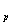in the unit disk

• F. and M. Riesz Theorem.
• Poisson representation for functions of class H.
• Blaschke products factorization.
• Classes H(3)  Hilbert Transform

• Hilbert Transform in L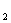.
• Hilbert Transform in Land M. Riesz Theorem.

(4)  Interpolation

• Carleson Measure.
• Carleson Theorem.

(5)  Functions of Bounded mean Oscillations

• Functions of bounded mean oscillations.
• John-Nirenberg Theorem.

Textbook:
Paul Koosis, Introduction to Hspaces, with an appendix on Wolff’s proof of the corona
theorem Cambridge University Press, 1980.

MAT 1011HS
INTRODUCTION TO LINEAR OPERATORS
G.A. Elliott

The course will survey the branch of mathematics developed (in its abstract form) primarily in the twentieth century and referred to variously as functional analysis, linear operators in Hilbert space, and operator algebras, among other names (for instance, more recently, to reflect the rapidly increasing scope of the subject, the phrase non-commutative geometry has been introduced). The intention will be to discuss a number (as many as conveniently possible) of the topics in Pedersen's textbook Analysis Now, reviewing more quickly those items with which people are familiar. Students will be encouraged to lecture on some of the material, and also work through (at least some of) the exercises in the book.
Prerequisites: Elementary analysis and linear algebra (including the spectral theorem for self-adjoint matrices)

Textbook
:
Gert K. Pedersen, Analysis Now, Revised Printing, Graduate Texts in Mathematics, Springer, 1989

Recommended references:
Paul R. Halmos, A Hilbert Space Problem Book, Second Edition, Graduate Texts in Mathematics, Springer, 1982
Mikael Rørdam, Flemming Larsen, and Niels J. Laustsen, An Introduction to K-Theory for C*-Algebras, London Mathematical Society Student Texts 49, Cambridge University Press, 2000

MAT 1045HF
PROBABILITY AND GEOMETRY ON GROUPS
G. Pete

Probability is one of the fastest developing areas of mathematics today, finding new connections to other branches constantly. One example is the rich interplay between large-scale geometric properties of a space and the behaviour of stochastic processes (like random walks and percolation) on the space. The obvious best source of discrete metric spaces are the Cayley graphs of finitely generated groups, especially that their large-scale geometric (and hence, probabilistic) properties reflect the algebraic properties. A famous example is the construction of expander graphs using group representations, another one is Gromov's theorem on the equivalence between a group being almost nilpotent and the polynomial volume growth of its Cayley graphs. The course will contain a large variety of interrelated topics in this area, with an emphasis on open problems.

Prerequisites: The core courses Real Analysis and Algebra are recommended. Former exposure to probability (e.g., the graduate probability courses) would be helpful.

Some references we will use:

S. Hoory, N. Linial and A. Wigderson:  Expander graphs and their applications,
Bulletin of AMS, 2006,
http://www.cs.huji.ac.il/~nati/PAPERS/expander_survey.pdf

M. Kapovich: Lectures on geometric group theory,
http://www.math.ucdavis.edu/~kapovich/EPR/ggt.pdf

A. Lubotzky: Discrete groups, expanding graphs and invariant measures,
Progress in Math. 125, Birkhauser Verlag, Basel, 1994.

R. Lyons with Y. Peres: Probability on trees and networks,
http://mypage.iu.edu/~rdlyons/prbtree/prbtree.html

W. Woess: Random walks on infinite graphs and groups, Cambridge University Press, 2000.

MAT 1062HF
INTRODUCTION TO GEOMETRIC FLOWS
I.M. Sigal

In this course we will study mean curvature, Ricci and harmonic map flows. We also plan to describe the curvature flow of networks of plane curves. We will give careful definitions of these flows, present existence results and results on formation of singularities (e.g. collapse to a point and neck-pinching) and soliton dynamics. We will also introduce main techniques, such as parabolic existence theory, maximum principles and monotonicity (entropy) formulae.

We will explain all needed notions from Differential Geometry and Partial Differential Equations, but knowledge of these subjects at an introductory level is required for this course.

References:

K. Ecker: Regularity theory for mean curvature flow, Birkhaeuser, 2004; ISBN 08 176 32433

P. Topping, Lectures on the Ricci flow, London Math Society Lecture Notes, series 325, Cambridge Univ. Press, 2006; ISBN 0-521-68947-3.

MAT 1063HF
INTRODUCTION TO MICROLOCAL ANALYSIS
F. Rochon

The following topics will be covered: Tempered distributions and the Fourier transform,
Pseudodifferential operators on the Euclidean space and their basic  properties,  elliptic regularity, Microlocalization and the wave front set, Pseudodifferential operators on manifolds, Application to the wave equation (propagation of singularities theorem), complex power and the residue trace.

Prerequisites:  Real Analysis (MAT1000HF and MAT1001HS)

Textbook:
Introduction to Microlocal Analysis, by Richard Melrose (available online: http://www-math.mit.edu/~rbm/iml90.pdf)

MAT 1120HF
LIE GROUPS AND CLIFFORD ALGEBRAS
E. Meinrenken

The plan of this course is to present the basic theory of Clifford algebras, with applications to Lie groups and Lie algebras. Detailed lecture notes will be provided. Topic include:

• Clifford algebras
• Spinor modules
• Dirac operators
• Lie groups
• Applications: Poincare-Birkhoff-Witt theorem, Duflo theorem, Weyl character formula, Borel-Weil theorem, multiplets of representations,...

Prerequisites: Basic knowledge of linear algebra and differential geometry (vector fields, differential forms).

References:
S. Sternberg: Lecture notes on Lie algebras. Available at
http://www.math.harvard.edu/people/SternbergShlomo.html
C. Chevalley: The algebraic theory of spinors and Clifford algebras (reprinted version), Springer 1997.
B. Lawson, L. Michelson: Spin geometry, Princeton University Press (1989).

MAT 1124HS
RANDOM MATRIX THEORY
B. Virag

Snapshots of random matrix theory and its numerous applications. Topics include limiting eigenvalue distributions, connections to exclusion processes, free probability, orthogonal polynomials, random Schrodinger operators, random polynomials and the Riemann zeta function. Feynmann diagrams and random planar graphs. Dyson's Brownian motion.

MAT 1155HF (MAT 448H1F)
INTRODUCTION TO ALGEBRAIC GEOMETRY AND COMMUTATIVE ALGEBRA
J. Kamnitzer

We will study the geometry of algebraic varieties over algebraically closed fields.  The course will cover affine varieties, nullstellensatz, projective varieties, Zariski topology, sheaves of functions and modules, dimension, smoothness, and line bundles.  All necessary commutative algebra results and concepts will be introduced and explained.

Textbook:
Daniel Perrin, Algebraic geometry, an introduction (Springer)

MAT 1197HF
INTRODUCTION TO AUTOMORPHIC FORMS
J. Arthur

The theory of automorphic forms has enjoyed remarkable progress in recent years. While the subject still has a long way to go, it is starting to realize some of its promise as a grand scheme to characterize basic objects from the arithmetic world and other areas of mathematics. The  course will be a broad introduction to automorphic forms, automorphic  representations and the Langlands programme.

It will have no formal prerequisites, other than a knowledge of the  material in the core courses, and will be at the level of an advanced  second year graduate course. Any of the Department's courses on Lie  groups, Lie algebras, modular forms, algebraic number theory or  representation theory of p-adic groups will provide very useful  background, but will not be essential. I will try to tailor the course to  what is most suitable for the audience.

Possible references:
J. Cogdell, H. Kim and R. Murty, Lectures on Automorphic L-functions,  Fields Institute Monographs, vol. 5, 2004
S. Gelbart, An elementary intoduction to the Langlands program, Bulletin  of the AMS, vol. 10, 1984, pp. 177-219
T. Bailey and A. Knapp (editors), Representation Theory and Automorphic  Forms, Proceedings of Symposia of Pure Mathematics, AMS, vol. 61, 1997

MAT 1198HS
AUTOMORPHIC FORMS AND THE TRACE FORMULA
J. Arthur

This would be a continuation of the fall course above. The trace formula is one of the most powerful tools in the theory of automorphic forms. Some simple cases will be a part of the first course, but in the second half here, we shall study the general trace formula. We shall see that its terms serve as windows to fundamental questions in harmonic analysis and representation theory, and their application to the Langlands programme.

References:
S. Gelbart, Lectures on the Arthur-Selberg trace formula, University Lecture Series, AMS, 1995
J. Arthur, An introduction to the trace formula, in Clay Mathematics Proceedings, AMS, 2005, pp. 1-263

MAT 1200HF
MODULAR FORMS AND HALF-INTEGRAL WEIGHT
V.K. Murty

Modular forms of half-integral weight arise naturally (for example, from the study of theta functions). In this introductory course, we shall study Hecke theory and L-functions associated to such modular forms, including the formula of Waldspurger.

MAT 1210HS
THE ARITHMETIC THEORY OF MODULAR CURVES
S. Kudla

This course will provide an introduction to the arithmetic theory of modular curves and the modular forms that live on them.

First we will cover the basic theory:

• the modular curves X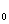(N), X(N), etc.
• modular forms, q-expansions, Hecke operators, newforms and oldforms,
• Hecke L-functions and their analytic properties.

We will continue to more advanced topics, possibly including:

• elliptic curves and complex multiplication,
• the field of modular functions and Shimura's reciprocity law
• Eisenstein series, Fourier expansions,
• the Kronecker limit formula and Chowla-Selberg formula, elliptic units,
• Eichler-Shimura theory, periods, rational structures
• the Jacobian varieties J(N)
• Rankin-Selberg L-functions and their special values

References:
J.-P. Serre, A course in arithmetic
G. Shimura, Introduction to the arithmetic theory of automorphic forms.
S. Lang, Introduction to modular forms.
C.L. Siegel, Advanced analytic number theory.

Prerequisites:
A basic knowledge of complex analysis, some algebraic number theory and some algebraic geometry.

MAT 1299HS
GENERAL TOPOLOGY
F. Tall

This course will cover many of the principal themes of General Topology. In contrast to the course I offered last year, there is no set-theoretic prerequisite. In fact, the only prerequisite is a nodding acquaintance with basic topological concepts, such as one would get from an undergraduate topology course. Among other topics, the course will cover:
i.   Compactness and generalizations,
ii.  Covering properties,
iii. Metrizability and generalized metric spaces,
iv. Completeness properties,
v.  Cardinal functions.

Throughout, there will be an emphasis on open problems. Material will be drawn from various sources, including:
1. R. Engelking, General Topology,
2. The articles on covering properties, generalized metric spaces, cardinal functions, and normality of products in the Handbook of Set-theoretic Topology,
3. The Encyclopedia of General Topology,
4. I. Juhasz, Cardinal Functions in Topology.

The choice of material is somewhat flexible, and can be varied depending on the background of the students. Typically in General Topology one deals with concepts such as compactness and metrizability that are familiar from the real line, and generalizes them in arbitrary topological spaces. Mindless generalizations abound; the trick is to find the right generalizations that illuminate deep structure and reveal significant questions to be investigated.

MAT 1302HS (APM 461H1S/CSC 2413HS)
COMBINATORIAL METHODS
B. Szegedy

A selection of topics from such areas as graph theory, combinatorial algorithms, enumeration, construction of combinatorial identities.

Prerequisite: MAT 224H1, recommended preparation MAT 344H1

MAT 1312HF
COMPUTABILITY AND COMPLEXITY IN GEOMETRY, TOPOLOGY, AND DYNAMICS
A. Nabutovsky and M. Yampolsky
(offered by the Fields Institute as part of the Thematic Program on the Foundations of Computational Mathematics)
Part I: Computability and Complexity in Topology and Differential Geometry
(A. Nabutovsky)
Computability (Turing machines, recursive functions, degrees of unsolvability), algorithmic information theory, unsolvable problems in group theory, group homology, non-computability in topology, applications of non-computability and algorithmic information theory in geometric calculus of variations (``thick" knots, local minima of Riemannian functionals, geometry of moduli spaces arising in differential geometry).
Literature: S. Weinberger, ``Computers, Rigidity and Moduli", Princeton University Press, 2005 and papers of the instructor.

Prerequisites
: Core graduate course in Topology.  Familiarity with foundations of Riemannian Geometry is desirable but not necessary.

Part II: Computability and Complexity in Dynamics (M. Yampolsky)

Basic notions of computable analysis: computable real functions, computable sets in R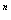. Dynamics of rational maps of the Riemann sphere: Fatou and Julia sets. Computability and complexity of Julia sets. Algorithms used to draw Julia sets versus theoretical complexity bounds. Constructing non-computable examples of Julia sets. Computability of filled Julia sets of polynomials.  Computable properties and the topology of Julia sets. Open problems.

Literature
: M. Braverman, M. Yampolsky, "Computability of Julia sets", Springer, 2008

MAT 1340HF (MAT 425H1F)
INTRODUCTION TO SMOOTH TOPOLOGY AND MAPPING DEGREE THEORY
A. Khovanskii

• Introduction to Smooth Topology: smooth manifolds and smooth mappings, inverse function theorem, Sard's theorem and transversality theorem.
• Mapping degree theory mod 2 and its applications: Brower theorem, linking number mod 2, Jordan theorem, oriented double covering, elementary topology of real algebraic curves in RP2.
• Mapping degree theory and its applications: Formula for the degree of a rational map f: RP1®RP1. Topological proofs of: the fundamental theorem of algebra, Sturm theorem about number of real roots, Tarski theorem about semi-algebraic sets, multi-dimensional Bezout theorem,
• Further applications: Hilbert polynomial for 0-dimensional algebraic sets, Euler-Jacobi formula, Eisenbud-Levin formula for index of isolated fix point of a vector field, topology of algebraic curves in RP2 (related to Hilbert's 16th problem), Petrovski inequalities.

References: J. Milnor: Topology from the Differential Viewpoint, a few original
papers will be suggested as additional material.

MAT 1341HS
MORSE THEORY
M. Gualtieri

This graduate course will be an introduction to the broad topic of Morse theory. We begin with the classical approach to Morse theory, studying the topology of manifolds using functions defined on them, and then move on to the modern formulations of Bott, Smale, Witten, and Floer, and explore some of the modern applications, which touch upon several fields of intense current study. Topics will include:

• Existence of Morse Functions
• Surgery, Handle Attachment, and Cobordisms
• Morse Inequalities
• Morse-Floer Homology
• Morse-Smale Dynamics
• Witten's approach to Morse theory
• Complex Morse theory (Picard-Lefschetz)
• Lagrangian Floer Homology and Pseudo-Holomorphic curves

Relevant textbooks and sources:

• “Morse Theory", Milnor.
• “Morse theory indomitable", Bott.
• “Supersymmetry and Morse theory", Witten.
• “Lectures on Morse Homology", Banyaga and Hurtubise.
• “An Invitation to Morse Theory",Nicolaescu.
• “Witten's complex and infinite-dimensional Morse theory", Floer.

Prerequisites: The prerequisites are an understanding of the geometry of smooth manifolds, homology and cohomology, vector fields, and Sard's theorem (Mat327H1 or Mat425H1 or MAT427H1 or 464H1 or, ideally, the first term of 1300Y - any of these would be acceptable prerequisites.)

MAT 1342HF (MAT 464H1F)
DIFFERENTIAL GEOMETRY
V. Kapovitch

Riemannian metrics, Levi-Civita connection, geodesics, curvature, Gauss equations, convexity, Complete manifolds and Hopf-Rinow theorem, Jacobi fields, Rauch comparison and variations of energy.

TextBook:  "Riemannian geometry" by Do Carmo.

Prerequisites: Manifolds, differential forms, group theory, basic algebraic topology (fundamental groups)

MAT 1345HF
FIBRE BUNDLES, CHARACTERISTIC CLASSES, AND K-THEORY
P. Selick

Topics may include: bundles and bundle operations; topological groups and group actions; classifying spaces; homotopy fibre; loop spaces; Stiefel-Whitney classes and Chern classes; other characteristic classes; axioms for generalized cohomology; K-theory; cohomology operations and Adams operations; Borel construction and equivariant cohomology.

References:
Milnor and Stasheff: "Characteristic Classes", Princeton University Press
Husemoller: "Fibre Bundles", Springer Graduate Texts in Mathematics
Atiyah: "K-Theory", Oxford University Press, ISBN: 978-0-19-853276-7
Selick: "Introduction to Homotopy Theory", Fields Institute Monograph Series

Prerequisites: Basic algebraic topology: particularly cohomology.

MAT 1347HS
HAMILTONIAN GROUP ACTIONS
Y. Karshon

This course is about Hamiltonian actions of compact Lie groups on symplectic manifolds.  Applications include using polytopes and graphs to read “classical" geometric information (volumes; symplectic packings) and “quantum" information (dimension/multiplicities of group representations).

Prerequisites: Manifolds, differential forms, and (co)homology. Background on Lie groups and symplectic geometry will be useful.

Relevant books:
Michele Audin, "Torus actions on symplectic manifolds".
Victor Guillemin, Eugene Lerman, and Shlomo Sternberg, "Symplectic Fibrations and Multiplicity Diagrams".
Viktor Ginzburg, Victor Guillemin, and Yael Karshon, "Moment Maps, Cobordisms, and Hamiltonian Group Actions".

MAT 1350HF
ALGEBRAIC KNOT THEORY
D. Bar-Natan

Agenda: Understand the promise and the difficulty of the not-yet-existent “Algebraic Knot Theory”.

An “Algebraic Knot Theory” should consist of two ingredients:

1. A map taking knots to algebraic entities; such a map may be useful, say, to tell different knots apart.
2. A collection of rules of the general nature of “if two knots are related in such and such a way, their corresponding algebraic entities are related in such and such a way”.  Such rules may allow us, say, to tell how far a knot is from the unknot or how far are two knots from each other.

(If you have seen homology in algebraic topology, recall that its strength stems from it being a functor.  Not merely it assigns groups to spaces, but further, if spaces are related by maps, the corresponding groups are related by a homomorphism.  We seek the same, or similar, for knots.)

The first ingredient for an “Algebraic Knot Theory” exists in many ways and forms; these are the many types and theories of “knot invariants”.  There is very little of the second ingredient at present, though when properly generalized and interpreted, the so-called Kontsevich Integral seems to be it.  But viewed from this angle, the Kontsevich Integral is remarkably poorly understood.

The purpose of this class is to understand all of the above.

MAT 1360HS
COMPLEX MANIFOLDS
J. Bland

An introduction to complex manifolds: vector bundles, complex line bundles, Hermitian connections, curvature, Kahler metrics, Kodaira embedding theorem, Hodge theory.

References:
Kodaira: ``Complex manifolds and Deformation of complex structures''
Griffiths and Harris: ``Principles of Algebraic Geometry''

Prerequisites:
A good background in differentiable manifolds including the de Rham complex of differential forms, Stoke's thereom, Frobenius integrability, and a good background in complex analysis in one variable.

MAT 1404HF (MAT 409H1F)
SET THEORY
F.D. Tall

We will introduce the basic principles of cardinals, ordinals, axiomatic set theory, infinitary combinatorics, consistency and  independence of the continuum hypothesis. In addition to the pure theory, the aim is to introduce students to the basic set-theoretic techniques applicable to other mathematical fields in which infinite sets play an essential role.

Prerequisite: an introductory real analysis course such as MAT 357H

References:
W. Just and M. Weese: Discovering Modern Set Theory, I and II, AMS.
K. Kunen: Set Theory, Elsevier.

MAT1508HS (APM446H1S)
APPLIED NONLINEAR EQUATIONS

I.M. Sigal

In this course we study partial differential equations appearing in Physics, Material Sciences, Biology and Differential Geometry. We will touch upon questions of existence, long-time behaviour, formation of singularities, pattern formation. We will also address questions of existence of static, traveling wave and localized solutions and their stability. The course will be relatively self-contained, but familiarity with elementary ordinary and partial differential equations and Fourier analysis will be assumed.

MAT 1700HS (APM 426H1S)
GENERAL RELATIVITY
R. Jerrard

Special relativity. The geometry of Lorentz manifolds. Gravity as a manifestation of spacetime curvature. Einstein's equation. Cosmological consequences: the big bang and inflationary universe. Schwarschild stars: the bending of light and perihelion procession of Mercury. Black hole dynamics. Gravitational waves.

Prerequisites: Thorough knowledge of linear algebra and multivariable calculus.

Reference: R. Wald, General Relativity, University of Chicago Press

MAT 1723HF (APM 421H1F)
QUANTUM MECHANICS
I.M. Sigal

The goal of this course is to explain key concepts of Quantum Mechanics and to arrive quickly to some topics which are at the forefront of active research. In particular we will present an introduction to quantum information theory, which has witnessed an explosion of research in the last decade and which involves deep and beautiful mathematics.

We will try to be as self-contained as possible and rigorous whenever the rigour is instructive. Whenever the rigorous treatment is prohibitively time-consuming we give an idea of the proof, if such exists, and/or explain the mathematics involved without providing all the details.

Prerequisites for this course: some familiarity with elementary ordinary and partial differential equations. Knowledge of elementary theory of functions and operators would be helpful.

Syllabus:

• Schroedinger equation
• Quantum observables
• Spectrum and evolution
• Density matrix
• Bose-Einstein condensation
• Quasiclassical asymptotics
• Approximate methods
• Hartree-Fock theory
• Open systems and Lindblad evolution
• Quantum entropy
• Quantum channels and information processing
• Quantum Shannon theorems

References:
S. Gustafson and I. M. Sigal, Mathematical Concepts of Quantum Mechanics,
2nd edition, Springer, 2005

For material not contained in this book, e.g. quantum information theory, we will try to provide handouts and refer to on-line sources.  Useful, but optional, books on the subject are

"Michael A. Nielsen and Isaac L. Chuang, Quantum Computation and Quantum
Information (Paperback - Sep 2000), Cambridge University Press, ISBN 0 521 63503 9 (paperback);

A. S. Holevo, Statistical Structure of Quantum Theory, Springer, 2001;

A. S. Holevo, Probabilistic and Statistical Aspects of Quantum Theory, Amsterdam, The Netherlands: North Holland.

MAT 1846HF
STATISTICAL MECHANICS AND BILLIARDS
D. Szasz

The basic philosophy of statistical physics is that its macroscopic laws arise from microscopic principles of newtonian mechanics. For their derivation billiard models have become most successful. These billiards are hyperbolic dynamical systems with singularities whose mathematical theory, however, is quite involved. Therefore - rather than treating hyperbolic billiards in general - my goal in this course is twofold: on the one hand, I explain parts of their 'dynamical systems' theory on simple paradigm models and, on the other hand, I will deal with some probabilistic methods which can be applied or are mimicked when deriving the macroscopic laws for billiard type models. At each topic, I will also formulate recent results and open problems.

Topics to be treated will be selected from the following ones:

• Proving ergodicity for hyperbolic maps: the Hopf method for the algebraic automorphism of the torus (Arnold's cat map)
• Proving ergodicity for hyperbolic maps with singularities: The fundamental theorem for hyperbolic billiards for the singular version of Arnold's cat map.
• A ball-avoiding theorem and ergodicity for a semi-dispersing billiard; a sketch
• Controlled mixing and CHT for hyperbolic maps with singularities: Young's towers and Perron-Frobenius operator technique for the singular version of Arnold's cat map
• Local CLT for the singular version of Arnold's cat map
• Markov maps of the interval: existence of absolutely continuous invariant measure
• CLT for the locally perturbed simple symmetric random walk in dimension 2: bounded range (coupling method)
• CLT for the locally perturbed simple symmetric random walk in dimension 2: unbounded range
• Probability theory of random walks with internal states
• A mechanical model of Brownian motion: the Rayleigh gas

• MAT 1739HS
A MATHEMATICAL INTRODUCTION TO STATISTICAL MECHANICS
O. Lanford

The goal of this course is to present the fundamental principles and results of statistical mechanics, emphasizing the physical background, in a way accessible to a mathematically-oriented audience. To avoid distracting technicalities, I will concentrate on classical lattice systems. In particular, with regret, I will say nothing at all about quantum statistical mechanics.

Program: Thermodynamics of simple materials. General principles of classical statistical mechanics: Boltzmann weight and ensembles. Presentation of the principal models: classical particle systems, lattice gases, and spin systems. Thermodynamic limit of the microcanonical entropy. Legendre transformations and thermodynamic limits of the other partition functions. Microcanonical entropy and the large deviations formalism for extensive observables. One-dimensional systems. Spontaneous magnetization in the two-dimensional Ising model. Correlation functions and their high-temperature expansions. Correlation inequalities. Equilibrium states, the variational principle, and Gibbs states. In the unlikely event that there is time left: Rudiments of nonequilibrium statistical mechanics: BBGKY hierarchy and Boltzmann equation,

Prerequisites: Very little mathematical background beyond advanced calculus and undergraduate analysis is formally needed, although “mathematical maturity” is, as always, helpful. I will need to use the language of elementary functional analysis and of general measure theory – on the level of measures on countable products of finite sets – but no deep results from these fields.

Literature: I plan to make available my own notes, which should serve as the main reference for the lectures. An excellent – but notoriously concise – exposition of most of the topics to be covered can be found in D. Ruelle, Statistical mechanics: rigorous results, reprinted 1999 by World Scientific.

MAT 1856HS (APM 466H1S)
MATHEMATICAL THEORY OF FINANCE
L. Seco

Introduction to the basic mathematical techniques in pricing theory and risk management: Stochastic calculus, single-period finance, financial derivatives (tree-approximation and Black-Scholes model for equity derivatives, American derivatives, numerical methods, lattice models for interest-rate derivatives), value at risk, credit risk, portfolio theory.

Prerequisites:  APM 346H1, STA 347H1

FOUNDATIONS OF MATHEMATICAL FINANCE
M. Grasselli

In addition to the regular time slot (Wednesdays 9:30-12:15), this course will occasionally meet on Thursdays from 9:30 to 12:15, when guests will deliver a set of lectures as part of the course. The guests lecturers for this course are Marco Frittelli, Eckhard Platen and Stan Pliska, and the details will be announced soon.

• Portfolio selection problem
• Fundamental theorem of asset pricing
• Semimartingale theory
• Primal and dual utility optimization problems
• Risk measure

• INTEREST RATES AND CREDIT RISK
T. Hurd

In addition to the regular time slot (Wednesdays 1:30-4:15), this course will occasionally meet on Thursdays from 1:30 to 4:15, when guests will deliver a set of lectures as part of the course. The guest lecturers for this course are Tomas Bjork and Kay Giesecke. The details will be announced soon.

• Spot rate models
• Heath-Jarrow-Morton theory
• Libor market models
• Structural credit risk models
• Reduced form credit risk models
• Multifirm default and correlation modeling

• STA2111HF
J. Quastel

Random variables, expected value, independence, laws of large numbers, random walks, martingales, Markov chains.

Prerequisite: measure theory (may be taken at the same time) or permission of the instructor.

Reference:
Theory of Probability & Leonid B. Koralov & McGraw-Hill Ryerson

STA 2211HS

B. Virag

Weak convergence, central limit theorems, stable laws, infinitely divisible laws, ergodic theorems, Brownian motion.

Reference: TBA

### COURSE IN TEACHING TECHNIQUES

The following course is offered to help train students to become effective tutorial leaders and eventually lecturers. It is not for degree credit and is not to be offered every year.

MAT 1499HS
TEACHING LARGE MATHEMATICS CLASSES
J. Repka

The goals of the course include techniques for teaching large classes, sensitivity to possible problems, and developing an ability to criticize one's own teaching and correct problems.

Assignments will include such things as preparing sample classes, tests, assignments, course outlines, designs for new courses, instructions for teaching assistants, identifying and dealing with various types of problems, dealing with administrative requirements, etc.

The course will also include teaching a few classes in a large course under the supervision of the instructor. A video camera will be available to enable students to tape their teaching for later (private) assessment.

COURSES FOR GRADUATE STUDENTS FROM OTHER DEPARTMENTS